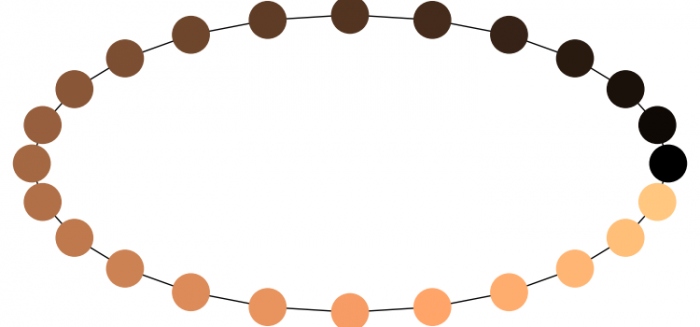# How to draw node colormap in NetworkX/Matplotlib?

To draw node colormap in matplotlib/netwokx, we can take the following steps −

• Set the figure size and adjust the padding between and around the subplots.
• Return the cycle graph $C_n$ of cyclically connected nodes.
• Position the nodes on a circle.
• Draw the graph G with Matplotlib.
• To display the figure, use show() method.

## Example

import matplotlib.pyplot as plt
import networkx as nx

plt.rcParams["figure.figsize"] = [7.50, 3.50]
plt.rcParams["figure.autolayout"] = True

G = nx.cycle_graph(24)

pos = nx.circular_layout(G)

nx.draw(G, pos, node_color=range(24), node_size=800, cmap='copper')

plt.show()

## Output